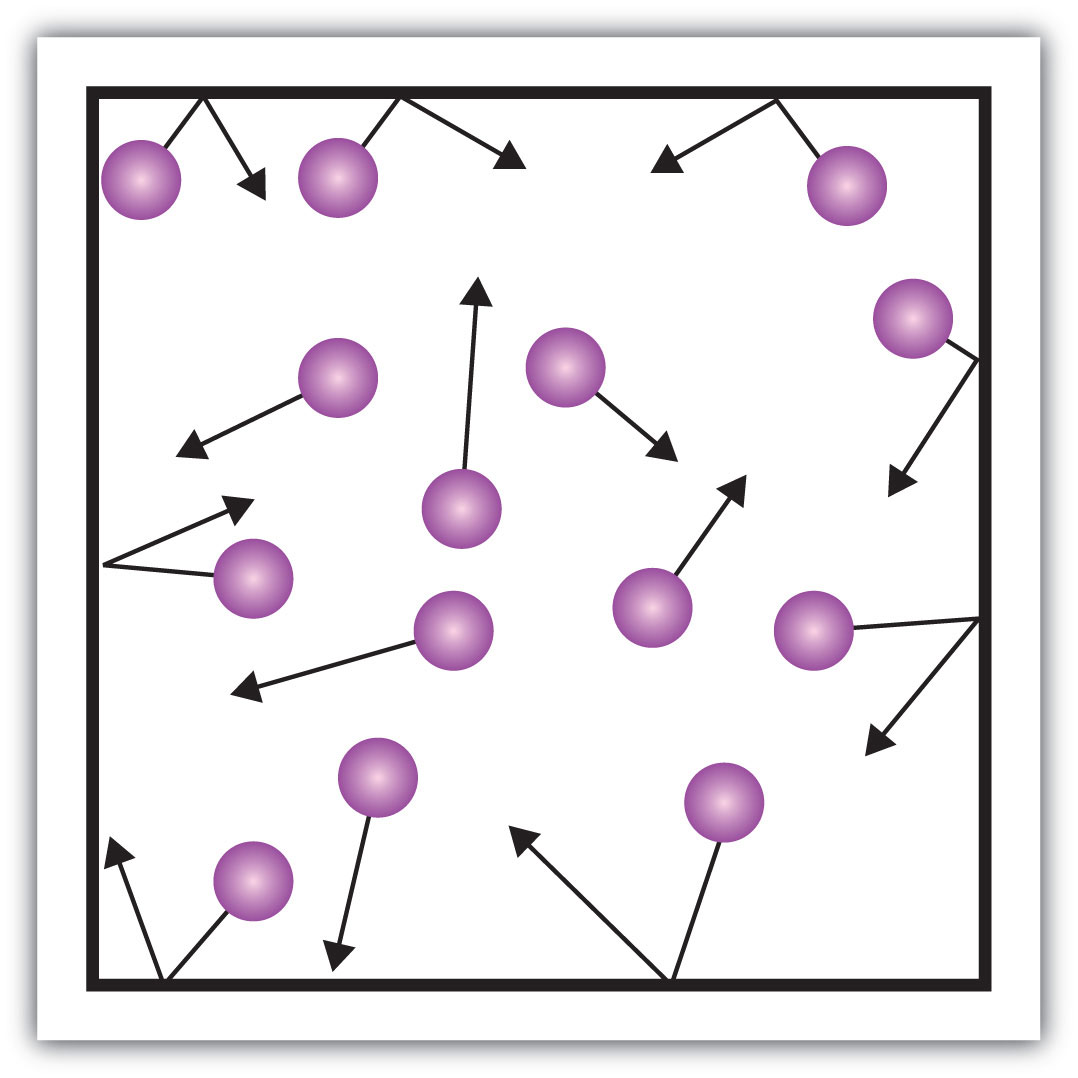## 8.3 Gases and Pressure

### Learning Objective

1. Describe the gas phase.

The gas phase is unique among the three states of matter in that there are some simple models we can use to predict the physical behavior of all gases—independent of their identities. We cannot do this for the solid and liquid states. In fact, the development of this understanding of the behavior of gases represents the historical dividing point between alchemy and modern chemistry. Initial advances in the understanding of gas behavior were made in the mid 1600s by Robert Boyle, an English scientist who founded the Royal Society (one of the world’s oldest scientific organizations).

How is it that we can model all gases independent of their chemical identity? The answer is in a group of statements called the kinetic theory of gasesThe fundamental theory of the behavior of gases.:

• Gases are composed of tiny particles that are separated by large distances.
• Gas particles are constantly moving, experiencing collisions with other gas particles and the walls of their container.
• The velocity of gas particles is related to the temperature of a gas.
• Gas particles do not experience any force of attraction or repulsion with each other.

Did you notice that none of these statements relates to the identity of the gas? This means that all gases should behave similarly. A gas that follows these statements perfectly is called an ideal gas. Most gases show slight deviations from these statements and are called real gases. However, the existence of real gases does not diminish the importance of the kinetic theory of gases.

One of the statements of the kinetic theory mentions collisions. As gas particles are constantly moving, they are also constantly colliding with each other and with the walls of their container. There are forces involved as gas particles bounce off the container walls (Figure 8.9 "Gas Pressure"). The force generated by gas particles divided by the area of the container walls yields pressureForce divided by area.. Pressure is a property we can measure for a gas, but we typically do not consider pressure for solids or liquids.

Figure 8.9 Gas PressurePressure is what results when gas particles rebound off the walls of their container.

The basic unit of pressure is the newton per square meter (N/m2). This combined unit is redefined as a pascalA unit of pressure equal to 1 newton of force per square meter of area. (Pa). One pascal is not a very large amount of pressure. A more useful unit of pressure is the barA unit of pressure equal to 100,000 Pa., which is 100,000 Pa (1 bar = 100,000 Pa). Other common units of pressure are the atmosphereA unit of pressure equal to the average atmospheric pressure at sea level. (atm), which was originally defined as the average pressure of Earth’s atmosphere at sea level; and mmHg (millimeters of mercury)A unit of pressure equal to the pressure generated by a column of mercury 1 mm high., which is the pressure generated by a column of mercury 1 mm high. The unit millimeters of mercury is also called a torrAnother name for millimeters of mercury., named after the Italian scientist Evangelista Torricelli, who invented the barometer in the mid-1600s. A more precise definition of atmosphere, in terms of torr, is that there are exactly 760 torr in 1 atm. A bar equals 1.01325 atm. Given all the relationships between these pressure units, the ability to convert from one pressure unit to another is a useful skill.

### Example 3

Write a conversion factor to determine how many atmospheres are in 1,547 mmHg.

Solution

Because 1 mmHg equals 1 torr, the given pressure is also equal to 1,547 torr. Because there are 760 torr in 1 atm, we can use this conversion factor to do the mathematical conversion:

Note how the torr units cancel algebraically.

### Skill-Building Exercise

1. Write a conversion factor to determine how many millimeters of mercury are in 9.65 atm.

The kinetic theory also states that there is no interaction between individual gas particles. Although we know that there are, in fact, intermolecular interactions in real gases, the kinetic theory assumes that gas particles are so far apart that the individual particles don’t “feel” each other. Thus, we can treat gas particles as tiny bits of matter whose identity isn’t important to certain physical properties.

### Concept Review Exercise

1. What is pressure, and what units do we use to express it?

1. Pressure is the force per unit area; its units can be pascals, torr, millimeters of mercury, or atmospheres.

### Key Takeaway

• The gas phase has certain general properties characteristic of that phase.

### Exercises

1. What is the kinetic theory of gases?

2. According to the kinetic theory of gases, the individual gas particles are (always, frequently, never) moving.

3. Why does a gas exert pressure?

4. Why does the kinetic theory of gases allow us to presume that all gases will show similar behavior?

5. Arrange the following pressure quantities in order from smallest to largest: 1 mmHg, 1 Pa, and 1 atm.

6. Which unit of pressure is larger—the torr or the atmosphere?

7. How many torr are there in 1.56 atm?

8. Convert 760 torr into pascals.

9. Blood pressures are expressed in millimeters of mercury. What would be the blood pressure in atmospheres if a patient’s systolic blood pressure is 120 mmHg and the diastolic blood pressure is 82 mmHg? (In medicine, such a blood pressure would be reported as “120/82,” spoken as “one hundred twenty over eighty-two.”)

10. In weather forecasting, barometric pressure is expressed in inches of mercury (in. Hg), where there are exactly 25.4 mmHg in every 1 in. Hg. What is the barometric pressure in millimeters of mercury if the barometric pressure is reported as 30.21 in. Hg?